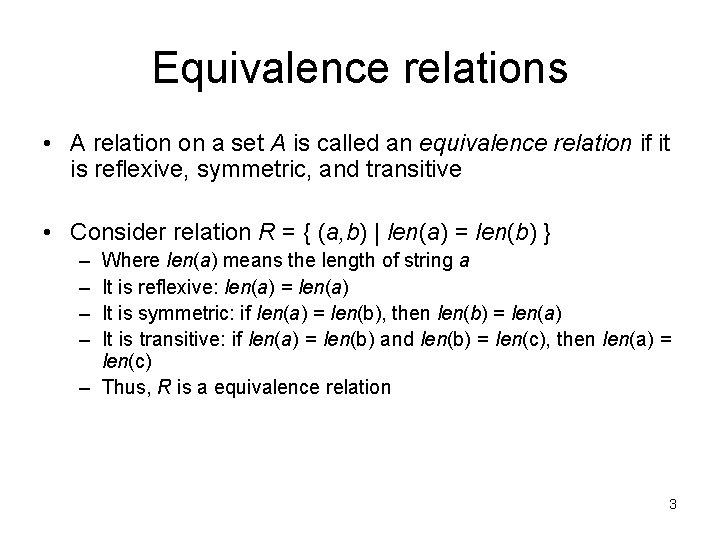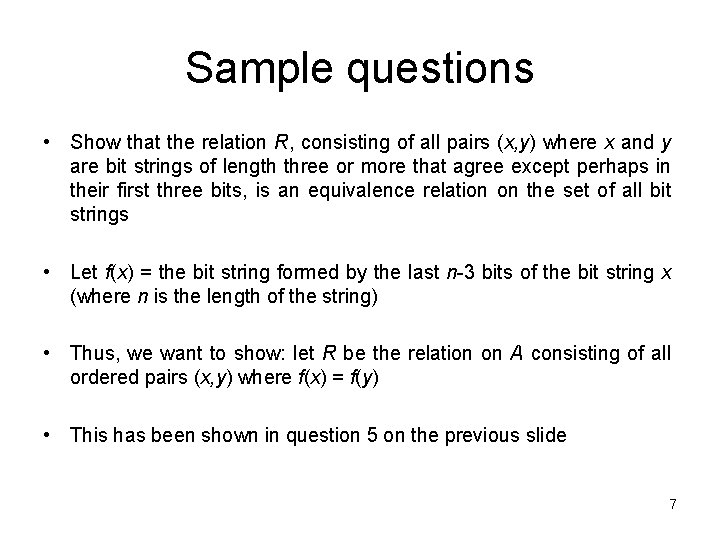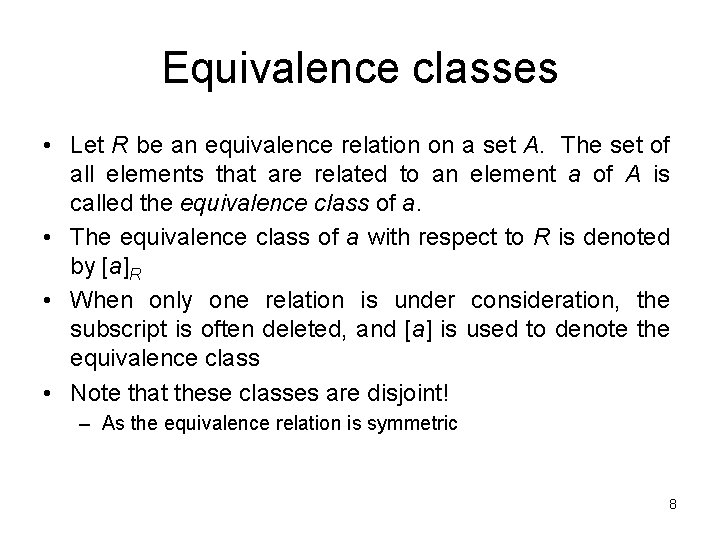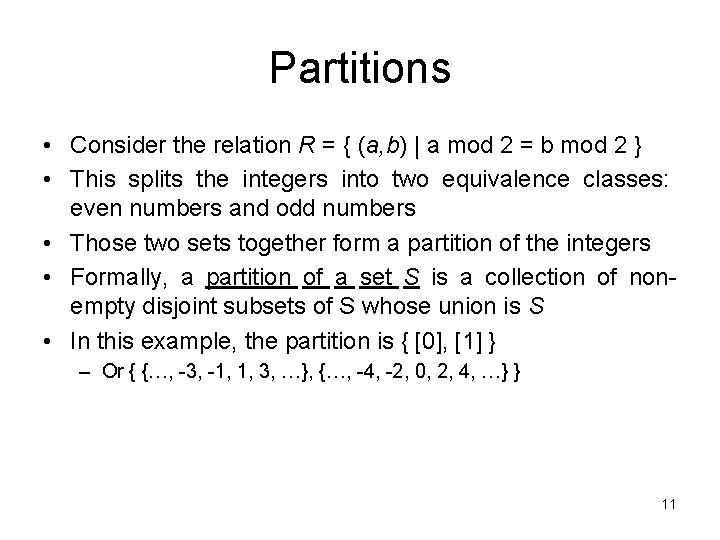# Equivalence Relations Aaron Bloomfield CS 202 Epp section

• Slides: 12
Download presentationEquivalence Relations Aaron Bloomfield CS 202 Epp, section ? ? ? 1Introduction • Certain combinations of relation properties are very useful – We won’t have a chance to see many applications in this course • In this set we will study equivalence relations – A relation that is reflexive, symmetric and transitive • Next slide set we will study partial orderings – A relation that is reflexive, antisymmetric, and transitive • The difference is whether the relation is symmetric or antisymmetric 2Equivalence relations • A relation on a set A is called an equivalence relation if it is reflexive, symmetric, and transitive • Consider relation R = { (a, b) | len(a) = len(b) } – – Where len(a) means the length of string a It is reflexive: len(a) = len(a) It is symmetric: if len(a) = len(b), then len(b) = len(a) It is transitive: if len(a) = len(b) and len(b) = len(c), then len(a) = len(c) – Thus, R is a equivalence relation 3Equivalence relation example • Consider the relation R = { (a, b) | m | a-b } – Called “congruence modulo m” • Is it reflexive: (a, a) R means that m | a-a – a-a = 0, which is divisible by m • Is it symmetric: if (a, b) R then (b, a) R – (a, b) means that m | a-b – Or that km = a-b. Negating that, we get b-a = -km – Thus, m | b-a, so (b, a) R • Is it transitive: if (a, b) R and (b, c) R then (a, c) R – – – (a, b) means that m | a-b, or that km = a-b (b, c) means that m | b-c, or that lm = b-c (a, c) means that m | a-c, or that nm = a-c Adding these two, we get km+lm = (a-b) + (b-c) Or (k+l)m = a-c Thus, m divides a-c, where n = k+l • Thus, congruence modulo m is an equivalence relation 4Sample questions • a) Which of these relations on {0, 1, 2, 3} are equivalence relations? Determine the properties of an equivalence relation that the others lack { (0, 0), (1, 1), (2, 2), (3, 3) } Has all the properties, thus, is an equivalence relation b) { (0, 0), (0, 2), (2, 0), (2, 2), (2, 3), (3, 2), (3, 3) } Not reflexive: (1, 1) is missing Not transitive: (0, 2) and (2, 3) are in the relation, but not (0, 3) c) { (0, 0), (1, 1), (1, 2), (2, 1), (2, 2), (3, 3) } Has all the properties, thus, is an equivalence relation d) { (0, 0), (1, 1), (1, 3), (2, 2), (2, 3), (3, 1), (3, 2) (3, 3) } Not transitive: (1, 3) and (3, 2) are in the relation, but not (1, 2) e) { (0, 0), (0, 1) (0, 2), (1, 0), (1, 1), (1, 2), (2, 0), (2, 2), (3, 3) } Not symmetric: (1, 2) is present, but not (2, 1) Not transitive: (2, 0) and (0, 1) are in the relation, but not (2, 1) 5Sample questions • Suppose that A is a non-empty set, and f is a function that has A as its domain. Let R be the relation on A consisting of all ordered pairs (x, y) where f(x) = f(y) – Meaning that x and y are related if and only if f(x) = f(y) • Show that R is an equivalence relation on A • Reflexivity: f(x) = f(x) – True, as given the same input, a function always produces the same output • Symmetry: if f(x) = f(y) then f(y) = f(x) – True, by the definition of equality • Transitivity: if f(x) = f(y) and f(y) = f(z) then f(x) = f(z) – True, by the definition of equality 6Sample questions • Show that the relation R, consisting of all pairs (x, y) where x and y are bit strings of length three or more that agree except perhaps in their first three bits, is an equivalence relation on the set of all bit strings • Let f(x) = the bit string formed by the last n-3 bits of the bit string x (where n is the length of the string) • Thus, we want to show: let R be the relation on A consisting of all ordered pairs (x, y) where f(x) = f(y) • This has been shown in question 5 on the previous slide 7Equivalence classes • Let R be an equivalence relation on a set A. The set of all elements that are related to an element a of A is called the equivalence class of a. • The equivalence class of a with respect to R is denoted by [a]R • When only one relation is under consideration, the subscript is often deleted, and [a] is used to denote the equivalence class • Note that these classes are disjoint! – As the equivalence relation is symmetric 8More on equivalence classes • Consider the relation R = { (a, b) | a mod 2 = b mod 2 } – Thus, all the even numbers are related to each other – As are the odd numbers • The even numbers form an equivalence class – As do the odd numbers • The equivalence class for the even numbers is denoted by  (or , or , etc. ) –  = { …, -4, -2, 0, 2, 4, … } – 2 is a representative of it’s equivalence class • There are only 2 equivalence classes formed by this equivalence relation 9More on equivalence classes • Consider the relation R = { (a, b) | a = b or a = -b } – Thus, every number is related to additive inverse • The equivalence class for an integer a: –  = { 7, -7 } –  = { 0 } – [a] = { a, -a } • There an infinite number of equivalence classes formed by this equivalence relation 10Partitions • Consider the relation R = { (a, b) | a mod 2 = b mod 2 } • This splits the integers into two equivalence classes: even numbers and odd numbers • Those two sets together form a partition of the integers • Formally, a partition of a set S is a collection of nonempty disjoint subsets of S whose union is S • In this example, the partition is { ,  } – Or { {…, -3, -1, 1, 3, …}, {…, -4, -2, 0, 2, 4, …} } 11Sample questions • Which of the following are partitions of the set of integers? a) The set of even integers and the set of odd integers Yes, it’s a valid partition b) The set of positive integers and the set of negative integers No: 0 is in neither set c) The set of integers divisible by 3, the set of integers leaving a remainder of 1 when divided by 3, and the set of integers leaving a remaineder of 2 when divided by 3 Yes, it’s a valid partition d) The set of integers less than -100, the set of integers with absolute value not exceeding 100, and the set of integers greater than 100 Yes, it’s a valid partition e) The set of integers not divisible by 3, the set of even integers, and the set of integers that leave a remainder of 3 when divided by 6 The first two sets are not disjoint (2 is in both), so it’s not a valid 12 partition